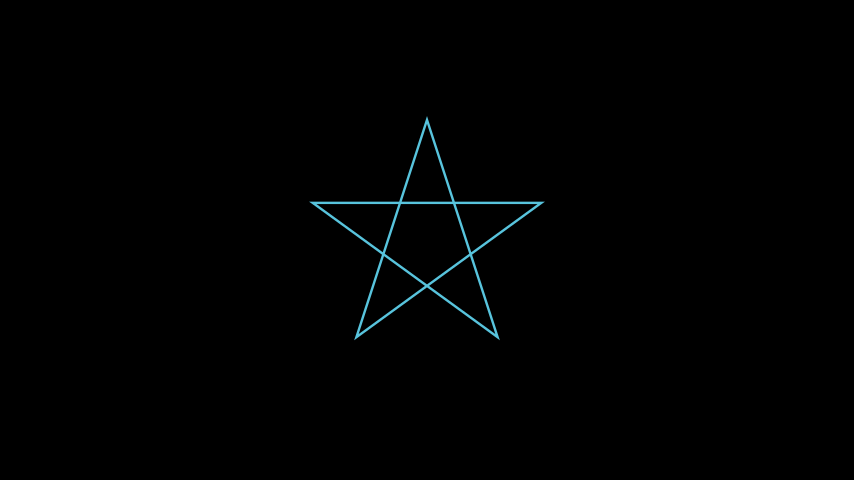# RegularPolygram#

Qualified name: `manim.mobject.geometry.polygram.RegularPolygram`

class RegularPolygram(num_vertices, *, density=2, radius=1, start_angle=None, **kwargs)[source]#

Bases: `Polygram`

A `Polygram` with regularly spaced vertices.

Parameters
• num_vertices (int) – The number of vertices.

• density (int) –

The density of the `RegularPolygram`.

Can be thought of as how many vertices to hop to draw a line between them. Every `density`-th vertex is connected.

• radius (float) – The radius of the circle that the vertices are placed on.

• start_angle (float | None) – The angle the vertices start at; the rotation of the `RegularPolygram`.

• kwargs – Forwarded to the parent constructor.

Examples

Example: RegularPolygramExample```from manim import *

class RegularPolygramExample(Scene):
def construct(self):
 `animate` Used to animate the application of any method of `self`. `animation_overrides` `color` `depth` The depth of the mobject. `fill_color` If there are multiple colors (for gradient) this returns the first one `height` The height of the mobject. `n_points_per_curve` `sheen_factor` `stroke_color` `width` The width of the mobject.